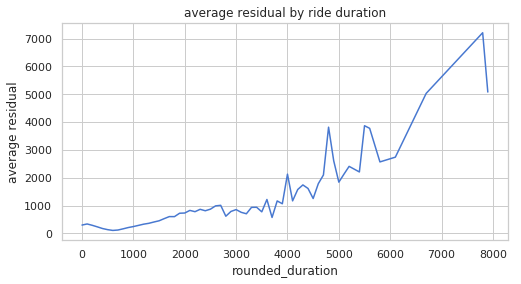# NYC Taxi Rides

In this project, I analyze New York City taxi data and make predictions on taxi ride duration times. The original data can be found here.

%matplotlib inline
import os
import pandas as pd
from datetime import datetime
import pandas as pd
from sklearn.model_selection import train_test_split
from sklearn.linear_model import LinearRegression, Ridge,BayesianRidge
from sklearn.cluster import MiniBatchKMeans
from sklearn.metrics import mean_squared_error
import sklearn.linear_model as lm
from math import radians, cos, sin, asin, sqrt
import seaborn as sns
import matplotlib
import numpy as np
import matplotlib.pyplot as plt

from pathlib import Path
from sqlalchemy import create_engine
from sklearn.model_selection import cross_val_score, train_test_split, GridSearchCV
sns.set(style="whitegrid", palette="muted")
from sqlalchemy import create_engine
from utils import timeit
plt.rcParams['figure.figsize'] = [16, 10]
plt.rcParams['font.size'] = 12
%matplotlib inline


# 1. The Data

!ls -lh /srv/db/taxi_2016_student_small.sqlite

-rw-r--r-- 1 root root 2.1G Nov 27 07:32 /srv/db/taxi_2016_student_small.sqlite

DB_URI = "sqlite:////srv/db/taxi_2016_student_small.sqlite"
TABLE_NAME = "taxi"

sql_engine = create_engine(DB_URI)
with timeit():
print(f"Table {TABLE_NAME} has {sql_engine.execute(f'SELECT COUNT(*) FROM {TABLE_NAME}').first()} rows!")

Table taxi has 15000000 rows!
1.00 s elapsed


Below we filter out taxi rides that occured during the big storm in January of 2016.

query1 = f"""
SELECT *
FROM {TABLE_NAME}
WHERE tpep_pickup_datetime
BETWEEN '2016-02-01' AND '2016-04-30'
AND record_id % 100 == 0
ORDER BY tpep_pickup_datetime
"""
with timeit():

3.06 s elapsed


Ignore this part below. We will use this later.

query2 = f"""
SELECT *
FROM {TABLE_NAME}
WHERE tpep_pickup_datetime
AND record_id % 100 == 0
ORDER BY tpep_pickup_datetime
"""
with timeit():

3.90 s elapsed


Aadding a duration column to the training dataset.

data['tpep_pickup_datetime'] = pd.to_datetime(data['tpep_pickup_datetime'])
data['tpep_dropoff_datetime'] = pd.to_datetime(data['tpep_dropoff_datetime'])

data['duration'] = data["tpep_dropoff_datetime"] - data["tpep_pickup_datetime"]
data['duration'] = data['duration'].dt.total_seconds()
data = data[data['duration'] < 12 * 3600]

data.shape

(75396, 21)

## 1.2 The Stats

Below is the statistical summary of the data.

data.describe()

record_id VendorID passenger_count trip_distance pickup_longitude pickup_latitude RatecodeID dropoff_longitude dropoff_latitude payment_type fare_amount extra mta_tax tip_amount tolls_amount improvement_surcharge total_amount duration
count 7.539600e+04 75396.000000 75396.000000 75396.000000 75396.000000 75396.000000 75396.000000 75396.000000 75396.000000 75396.000000 75396.000000 75396.000000 75396.000000 75396.000000 75396.000000 75396.000000 75396.000000 75396.000000
mean 2.849081e+07 1.530068 1.657595 2.929089 -72.853346 40.133951 1.074739 -72.891522 40.155681 1.340761 12.741845 0.338980 0.497238 1.786719 0.310740 0.299610 15.975131 821.843944
std 1.013156e+07 0.499098 1.307959 3.602263 9.035067 4.977352 1.908449 8.881745 4.892979 0.491848 11.071461 0.452275 0.039643 2.496170 1.441743 0.013728 13.627351 677.299095
min 1.090710e+07 1.000000 0.000000 0.000000 -77.807198 0.000000 1.000000 -77.039436 0.000000 1.000000 -52.000000 -4.500000 -0.500000 0.000000 0.000000 -0.300000 -57.300000 -16.000000
25% 1.971725e+07 1.000000 1.000000 1.000000 -73.991669 40.736443 1.000000 -73.991196 40.734812 1.000000 6.500000 0.000000 0.500000 0.000000 0.000000 0.300000 8.500000 391.000000
50% 2.847560e+07 2.000000 1.000000 1.700000 -73.981453 40.753426 1.000000 -73.979469 40.753880 1.000000 9.500000 0.000000 0.500000 1.350000 0.000000 0.300000 11.800000 654.000000
75% 3.729255e+07 2.000000 2.000000 3.110000 -73.966507 40.767995 1.000000 -73.962349 40.769169 2.000000 14.500000 0.500000 0.500000 2.360000 0.000000 0.300000 17.760000 1058.000000
max 4.643400e+07 2.000000 6.000000 55.600000 0.000000 44.932213 99.000000 0.000000 41.247791 4.000000 429.000000 4.500000 0.500000 160.000000 57.750000 0.300000 487.050000 37874.000000

## 1.3 Cleaning the Data

Everything looks pretty good; however, the duration stands out. The duration column is measured in seconds. The min is -16 seconds and the max is 149,481 seconds (41.5 hrs). Let's go ahead and remove all the taxi rides that have a negative duration because this is likely due to an error.

cleaned_data = data[data['duration'] >= 0]
cleaned_data = data[data['duration'] < 10000]
cleaned_data.shape

(75389, 21)

Next, we want filter out any taxi ride that is not within the bounds of the New York City region.

cleaned_data = cleaned_data[cleaned_data['pickup_longitude'] <= -73.75]
cleaned_data = cleaned_data[cleaned_data['pickup_longitude'] >= -74.03]
cleaned_data = cleaned_data[cleaned_data['pickup_latitude'] <= 40.85]
cleaned_data = cleaned_data[cleaned_data['pickup_latitude'] >= 40.63]
cleaned_data = cleaned_data[cleaned_data['dropoff_longitude'] <= -73.75]
cleaned_data = cleaned_data[cleaned_data['dropoff_longitude'] >= -74.03]
cleaned_data = cleaned_data[cleaned_data['dropoff_latitude'] <= 40.85]
cleaned_data = cleaned_data[cleaned_data['dropoff_latitude'] >= 40.63]
cleaned_data.shape

(73127, 21)

Also, the passenger_count column has a minimum of 0, which doesn't make sense. So now we remove all taxi rides with 0 passengers.

cleaned_data = cleaned_data[cleaned_data['passenger_count'] > 0]
cleaned_data.shape

(73126, 21)
num_records_removed = data.shape - cleaned_data.shape
proportion_records_removed = num_records_removed / data.shape
txt = f"""Number of records removed: {num_records_removed}
Proportion of records removed: {proportion_records_removed}"""
print(txt)

Number of records removed: 2270
Proportion of records removed: 0.030107698021115178


## Below we send the code away for part 4.

Path("data/final").mkdir(parents=True, exist_ok=True)
data_file = Path("data/final", "cleaned_data.hdf") # Path of hdf file
cleaned_data.to_hdf(data_file, "cleaned_data") # Train data of hdf file/


## 1.4 Test Train Split

train_df, test_df = train_test_split(cleaned_data, test_size=0.2, random_state=42)

train_df.shape, test_df.shape

((58500, 21), (14626, 21))

# 2. EDA on Taxi Data

Let's begin the EDA process by looking at ride duration in a histogram.

plt.hist(train_df['duration'], bins=90)
plt.xlabel('duration')
plt.ylabel('number of train records')
plt.show()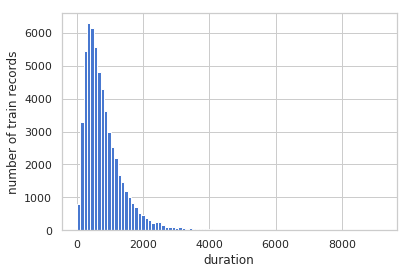Below I apply a log transformation to the data.

log_dur = np.log(train_df['duration'] + 1)
plt.hist(log_dur, bins=90)
plt.xlabel('log(duration)')
plt.ylabel('number of train records')
plt.show()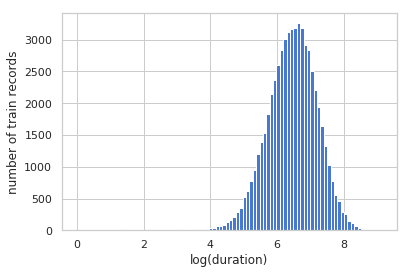train_df.loc[:,'tpep_pickup_datetime'] = pd.to_datetime(train_df['tpep_pickup_datetime'])
test_df.loc[:,'tpep_pickup_datetime'] = pd.to_datetime(test_df['tpep_pickup_datetime'])

train_df.loc[:, 'pickup_date'] = train_df.loc[:, 'tpep_pickup_datetime'].dt.date
test_df.loc[:, 'pickup_date'] = test_df.loc[:, 'tpep_pickup_datetime'].dt.date

train_df.loc[:, 'tpep_dropoff_datetime'] = pd.to_datetime(train_df.loc[:, 'tpep_dropoff_datetime'])
test_df.loc[:, 'tpep_dropoff_datetime'] = pd.to_datetime(test_df.loc[:, 'tpep_dropoff_datetime'])
all_data.loc[:,'tpep_pickup_datetime'] = pd.to_datetime(all_data['tpep_pickup_datetime'])

train_df.loc[:, 'day_of_week'] = train_df.loc[:, 'tpep_pickup_datetime'].dt.dayofweek
test_df.loc[:, 'day_of_week'] = test_df.loc[:, 'tpep_pickup_datetime'].dt.dayofweek

all_data.loc[:, 'pickup_date'] = all_data.loc[:, 'tpep_pickup_datetime'].dt.date
all_data.loc[:, 'tpep_dropoff_datetime'] = pd.to_datetime(all_data.loc[:, 'tpep_dropoff_datetime'])

/srv/conda/envs/data100/lib/python3.6/site-packages/pandas/core/indexing.py:543: SettingWithCopyWarning:
A value is trying to be set on a copy of a slice from a DataFrame.
Try using .loc[row_indexer,col_indexer] = value instead

See the caveats in the documentation: http://pandas.pydata.org/pandas-docs/stable/indexing.html#indexing-view-versus-copy
self.obj[item] = s
/srv/conda/envs/data100/lib/python3.6/site-packages/pandas/core/indexing.py:362: SettingWithCopyWarning:
A value is trying to be set on a copy of a slice from a DataFrame.
Try using .loc[row_indexer,col_indexer] = value instead

See the caveats in the documentation: http://pandas.pydata.org/pandas-docs/stable/indexing.html#indexing-view-versus-copy
self.obj[key] = _infer_fill_value(value)


## 2.2 Removing More Outliers

Next, let's look at the number of rides over time.

plt.figure(figsize=(9,3))
plt5 = plt.plot(all_data.groupby('pickup_date').count()[['record_id']], '-', label='train')
plt5 = plt.title('Trips over Time.')
plt5 = plt.legend(loc=0)
plt5 = plt.ylabel('Trips')
plt.show()

fig = plt5.get_figure()
fig.savefig('all_months.png')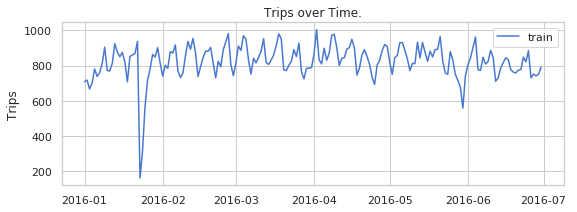As you can see in the plot above, the storm causes a massive drop in rides. This would throw off the accuracy of our model, so we want to bring that drop as close to the 'pipeline' of the data as possible as this is a pretty unusual experience. There is also a drop in late May 2016, which probably correlates to Memorial Day Weekend. Selecting dates from February to April avoids these outliers.

plt.figure(figsize=(15,6))
plt6 = plt.plot(train_df.groupby('pickup_date').count()[['record_id']], '-', label='train')
plt6 = plt.plot(test_df.groupby('pickup_date').count()[['record_id']], '-', label='test')
plt6 = plt.title('Trips From February to March')
plt6 = plt.legend(loc=0)
plt6 = plt.ylabel('Trips')
plt.show()

fig = plt6.get_figure()
fig.savefig('my_months.png')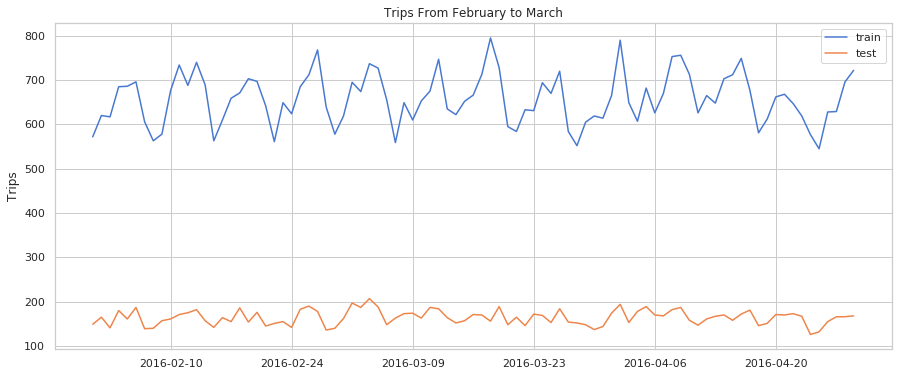It might also help to talk a look at ride duration vs day_of_week.

plt.figure(figsize=(12,4))
plt1 = sns.barplot(x='day_of_week', y='duration', data=train_df)
plt.suptitle("Duration of Rides per Day of Week")
plt.show()

fig = plt1.get_figure()
fig.savefig('duation of rides per day of week.png')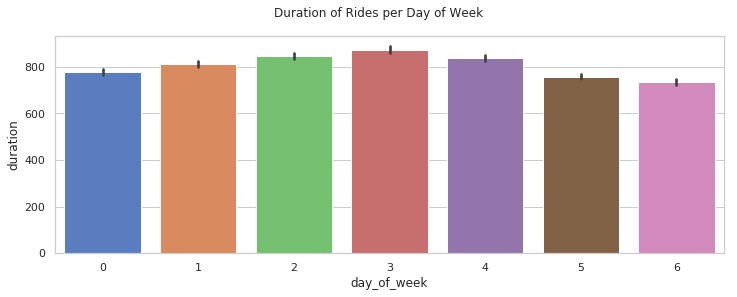# 3. Feature Engineering

Let's start by looking at different distance functions.

## 3.1 Distance

Below we define a couple distance methods we will be comparing.

# Copied from part 2
def haversine(lat1, lng1, lat2, lng2):
"""
Compute haversine distance
"""
lat1, lng1, lat2, lng2 = map(np.radians, (lat1, lng1, lat2, lng2))
lat = lat2 - lat1
lng = lng2 - lng1
d = np.sin(lat * 0.5) ** 2 + np.cos(lat1) * np.cos(lat2) * np.sin(lng * 0.5) ** 2
h = 2 * average_earth_radius * np.arcsin(np.sqrt(d))
return h

# Copied from part 2
def manhattan_distance(lat1, lng1, lat2, lng2):
"""
Compute Manhattan distance
"""
a = haversine(lat1, lng1, lat1, lng2)
b = haversine(lat1, lng1, lat2, lng1)
return a + b

# Copied from part 2
def bearing(lat1, lng1, lat2, lng2):
"""
Compute the bearing, or angle, from (lat1, lng1) to (lat2, lng2).
A bearing of 0 refers to a NORTH orientation.
"""
lat1, lng1, lat2, lng2 = map(np.radians, (lat1, lng1, lat2, lng2))
x = np.cos(lat1) * np.sin(lat2) - np.sin(lat1) * np.cos(lat2) * np.cos(lng_delta_rad)
return np.degrees(np.arctan2(y, x))

# Copied from part 2
"""
"""
df.is_copy = False # propogate write to original dataframe
df.loc[:, 'month'] = df['tpep_pickup_datetime'].dt.month
df.loc[:, 'week_of_year'] = df['tpep_pickup_datetime'].dt.weekofyear
df.loc[:, 'day_of_month'] = df['tpep_pickup_datetime'].dt.day
df.loc[:, 'day_of_week'] = df['tpep_pickup_datetime'].dt.dayofweek
df.loc[:, 'hour'] = df['tpep_pickup_datetime'].dt.hour
df.loc[:, 'week_hour'] = df['tpep_pickup_datetime'].dt.weekday * 24 + df['hour']
return df

# Copied from part 2
"""
"""
df.is_copy = False # propogate write to original dataframe
df.loc[:, 'manhattan'] = manhattan_distance(lat1=df['pickup_latitude'],
lng1=df['pickup_longitude'],
lat2=df['dropoff_latitude'],
lng2=df['dropoff_longitude'])

df.loc[:, 'bearing'] = bearing(lat1=df['pickup_latitude'],
lng1=df['pickup_longitude'],
lat2=df['dropoff_latitude'],
lng2=df['dropoff_longitude'])
df.loc[:, 'haversine'] = haversine(lat1=df['pickup_latitude'],
lng1=df['pickup_longitude'],
lat2=df['dropoff_latitude'],
lng2=df['dropoff_longitude'])
return df

def select_columns(data, *columns):
return data.loc[:, columns]

train_df = add_time_columns(train_df)

/srv/conda/envs/data100/lib/python3.6/site-packages/pandas/core/generic.py:4388: FutureWarning: Attribute 'is_copy' is deprecated and will be removed in a future version.
object.__getattribute__(self, name)
/srv/conda/envs/data100/lib/python3.6/site-packages/pandas/core/generic.py:4389: FutureWarning: Attribute 'is_copy' is deprecated and will be removed in a future version.
return object.__setattr__(self, name, value)

plt.figure(figsize=(12,4))
sns.lineplot(x='hour', y='haversine', data=train_df, label="Haversine")
sns.lineplot(x='hour', y='manhattan', data=train_df, label="Manhattan")
sns.lineplot(x='hour', y='trip_distance', data=train_df, label="trip_distance")
plt.suptitle("Distances per Hour of Day")
plt.legend()
plt.show()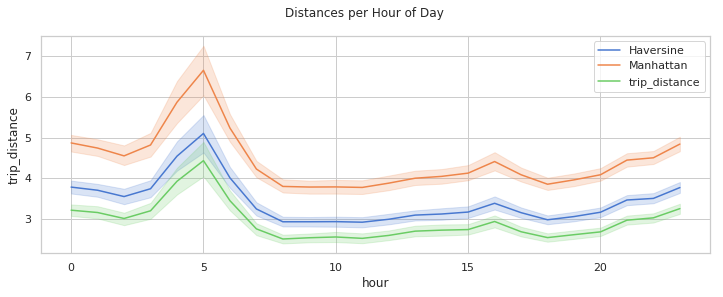Below I check to see if the price of the taxi ride has anything to do with its duration.

plt3 = sns.scatterplot(x='fare_amount', y='duration', data=train_df)
plt.show()

fig = plt3.get_figure()
fig.savefig('b4log.png')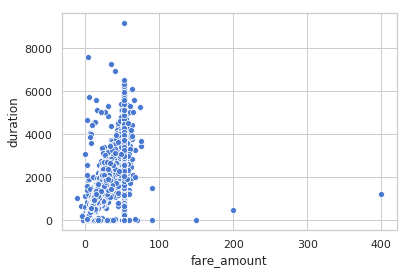This doesn't look too helpful, so below I look at the log of the price to see if we can get a more normal distribution.

train_df['fare_amount'] = np.log(np.abs(train_df['fare_amount']) + 1)
test_df['fare_amount'] = np.log(np.abs(test_df['fare_amount']) + 1)

train_df['total_amount'] = np.log(np.abs(train_df['total_amount']) + 1)
test_df['total_amount'] = np.log(np.abs(test_df['total_amount']) + 1)

plt4 = sns.scatterplot(x='fare_amount', y='duration', data=train_df)
plt.show()

fig = plt4.get_figure()
fig.savefig('afterlog.png')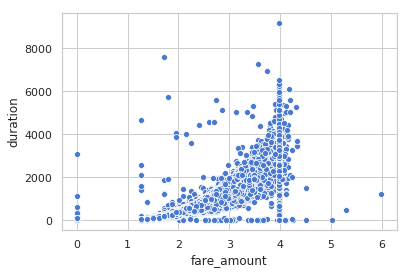train_end = train_df
test_end = test_df


## 3.2 One-Hot-Encoding

Let's search for some more features. As we saw in part 2, day_of_week may have something to do with ride durations. Now, I do some one-hot-encoding to select more features.

dow_train = pd.get_dummies(train_df['day_of_week'], prefix='dow', prefix_sep='_')
dow_test = pd.get_dummies(test_df['day_of_week'], prefix='dow', prefix_sep='_')

month_train = pd.get_dummies(train_df['month'], prefix='m', prefix_sep='_')
month_test = pd.get_dummies(test_df['month'], prefix='m', prefix_sep='_')

hour_train = pd.get_dummies(train_df['hour'], prefix='h', prefix_sep='_')
hour_test = pd.get_dummies(test_df['hour'], prefix='h', prefix_sep='_')

store_train = pd.get_dummies(train_df['store_and_fwd_flag'], prefix='h', prefix_sep='_')
store_test = pd.get_dummies(test_df['store_and_fwd_flag'], prefix='h', prefix_sep='_')


## 3.3 Combining the Data

Features we might want to use at are trip_distance, haversine, hour, month, day_of_week, and bearing (direction). Below, we get rid of all the categorical data that we will not use in our model.

# storing duration columns for later
train_response = train_df['duration']
test_response = test_df['duration']

train_df = train_df.drop(['record_id', 'VendorID', 'tpep_pickup_datetime',
'tpep_dropoff_datetime', 'passenger_count', 'trip_distance',
'pickup_longitude', 'pickup_latitude', 'RatecodeID',
'store_and_fwd_flag', 'dropoff_longitude', 'dropoff_latitude',
'payment_type', 'extra', 'mta_tax', 'tip_amount',
'tolls_amount', 'improvement_surcharge', 'duration',
'pickup_date', 'week_of_year', 'day_of_month', 'week_hour', 'month',
'day_of_week', 'hour'], axis=1)

test_df = test_df.drop(['record_id', 'VendorID', 'tpep_pickup_datetime',
'tpep_dropoff_datetime', 'passenger_count', 'trip_distance',
'pickup_longitude', 'pickup_latitude', 'RatecodeID',
'store_and_fwd_flag', 'dropoff_longitude', 'dropoff_latitude',
'payment_type', 'extra', 'mta_tax', 'tip_amount',
'tolls_amount', 'improvement_surcharge',
'pickup_date', 'week_of_year', 'day_of_month', 'week_hour', 'month',
'day_of_week', 'hour', 'duration'], axis=1)

print(train_df.shape)
print(test_df.shape)

(58500, 5)
(14626, 5)

# Combining dummies with trest and train dataset

Train_Master = pd.concat([train_df, dow_train, month_train, hour_train, store_train], axis=1)
Test_Master = pd.concat([test_df, dow_test, month_test, hour_test, store_test], axis=1)

print(Train_Master.shape)
print(Test_Master.shape)

(58500, 41)
(14626, 41)


# 4.1 Building a Model

Below, we create and define our model that predicts a taxi ride's duration.

## 4.1.1

linear_model = lm.LinearRegression(fit_intercept=True)
linear_model.fit(Train_Master, train_response)

LinearRegression(copy_X=True, fit_intercept=True, n_jobs=1, normalize=False)
pred_response = linear_model.predict(Train_Master)

def mae(actual, predicted):
"""
Calculates MAE from actual and predicted values
Input:
actual (1D array-like): vector of actual values
predicted (1D array-like): vector of predicted/fitted values
Output:
a float, the MAE
"""

mae = np.mean(np.abs(actual - predicted))
return mae

train_error = mae(train_response, linear_model.predict(Train_Master))
test_error = mae(test_response, linear_model.predict(Test_Master))
print("Training error: ", train_error)
print("Testing error: ", test_error)

Training error:  149.740987617
Testing error:  151.133119474


# Data Pipeline

I will now encapsulate my entire pipeline into a single function process_data_gm. gm is shorthand for "guided model".

def haversine(lat1, lng1, lat2, lng2):
"""
Compute haversine distance
"""
lat1, lng1, lat2, lng2 = map(np.radians, (lat1, lng1, lat2, lng2))
lat = lat2 - lat1
lng = lng2 - lng1
d = np.sin(lat * 0.5) ** 2 + np.cos(lat1) * np.cos(lat2) * np.sin(lng * 0.5) ** 2
h = 2 * average_earth_radius * np.arcsin(np.sqrt(d))
return h

# Copied from part 2
def manhattan_distance(lat1, lng1, lat2, lng2):
"""
Compute Manhattan distance
"""
a = haversine(lat1, lng1, lat1, lng2)
b = haversine(lat1, lng1, lat2, lng1)
return a + b

# Copied from part 2
def bearing(lat1, lng1, lat2, lng2):
"""
Compute the bearing, or angle, from (lat1, lng1) to (lat2, lng2).
A bearing of 0 refers to a NORTH orientation.
"""
lat1, lng1, lat2, lng2 = map(np.radians, (lat1, lng1, lat2, lng2))
x = np.cos(lat1) * np.sin(lat2) - np.sin(lat1) * np.cos(lat2) * np.cos(lng_delta_rad)
return np.degrees(np.arctan2(y, x))

# Copied from part 2
"""
"""
df.is_copy = False # propogate write to original dataframe
df.loc[:, 'month'] = df['tpep_pickup_datetime'].dt.month
df.loc[:, 'week_of_year'] = df['tpep_pickup_datetime'].dt.weekofyear
df.loc[:, 'day_of_month'] = df['tpep_pickup_datetime'].dt.day
df.loc[:, 'day_of_week'] = df['tpep_pickup_datetime'].dt.dayofweek
df.loc[:, 'hour'] = df['tpep_pickup_datetime'].dt.hour
df.loc[:, 'week_hour'] = df['tpep_pickup_datetime'].dt.weekday * 24 + df['hour']
return df

# Copied from part 2
"""
"""
df.is_copy = False # propogate write to original dataframe
df.loc[:, 'manhattan'] = manhattan_distance(lat1=df['pickup_latitude'],
lng1=df['pickup_longitude'],
lat2=df['dropoff_latitude'],
lng2=df['dropoff_longitude'])

df.loc[:, 'bearing'] = bearing(lat1=df['pickup_latitude'],
lng1=df['pickup_longitude'],
lat2=df['dropoff_latitude'],
lng2=df['dropoff_longitude'])
df.loc[:, 'haversine'] = haversine(lat1=df['pickup_latitude'],
lng1=df['pickup_longitude'],
lat2=df['dropoff_latitude'],
lng2=df['dropoff_longitude'])
return df

def select_columns(data, *columns):
return data.loc[:, columns]

def process_data_gm1(data, test=False):
X = (
data

# Transform data

.pipe(select_columns,
'pickup_longitude',
'pickup_latitude',
'dropoff_longitude',
'dropoff_latitude',
'manhattan',
)
)
if test:
y = None
else:
y = data['duration']

return X, y


I will use my pipeline defined above to pre-process my training and test data in exactly the same way. The functions make this relatively easy to do!

# Train
X_train, y_train = process_data_gm1(train_end)
X_val, y_val = process_data_gm1(test_end)
guided_model_1 = lm.LinearRegression(fit_intercept=True)
guided_model_1.fit(X_train, y_train)

# Predict
y_train_pred = guided_model_1.predict(X_train)
y_val_pred = guided_model_1.predict(X_val)

/srv/conda/envs/data100/lib/python3.6/site-packages/pandas/core/generic.py:4388: FutureWarning: Attribute 'is_copy' is deprecated and will be removed in a future version.
object.__getattribute__(self, name)
/srv/conda/envs/data100/lib/python3.6/site-packages/pandas/core/generic.py:4389: FutureWarning: Attribute 'is_copy' is deprecated and will be removed in a future version.
return object.__setattr__(self, name, value)


The resulting model really is a linear model, i.e. the predictions are simply generated by the product $\Phi\theta$. For example, the line of code below generates a prediction for $x_1$ by computing $\phi_1^T\theta$. Here guided_model_1.coef_ is $\theta$ and X_train.iloc[0, :] is $\phi_1$.

Note that here the dummy intercept term is not included in $\Phi$.

X_train.iloc[0, :].dot(guided_model_1.coef_) + guided_model_1.intercept_

545.48453622261877
y_train_pred

545.48453622258967

I will use Mean Absolute Error (MAE), a.k.a. mean L1 loss, to measure the quality of my model.

$$MAE =\frac{1}{n}\sum_{i} | y_i -\hat{y_i}|$$

Using domain knowledge that most rides are short in duration (median is roughly 600 seconds), we know that MSE is susceptible to outliers. Given that some of the outliers in our dataset are quite extreme, it is probably better to optimize for the majority of rides rather than for the outliers.

def mae(actual, predicted):
"""
Calculates MAE from actual and predicted values
Input:
actual (1D array-like): vector of actual values
predicted (1D array-like): vector of predicted/fitted values
Output:
a float, the MAE
"""

mae = np.mean(np.abs(actual - predicted))
return mae


## Visualizing Error

The error should be between 200 and 300 MAE, which means the model was off by roughly 3-5 minutes on trips of average length 12 minutes. This is fairly decent performance given that the basic model uses only using the pickup/dropoff latitude and manhattan distance of the trip. 3-5 minutes may seem like a lot for a trip of 12 minutes, but keep in mind that this is the average error. This metric is susceptible to extreme outliers, which exist in the dataset.

Now I will visualize the residual for the validation set and plot the following:

1. Distribution of residuals
2. Average residual grouping by ride duration
plt.figure(figsize=(8,4))
sns.distplot(np.abs(y_val - y_val_pred))
plt.xlabel('residual')
plt.title('distribution of residuals');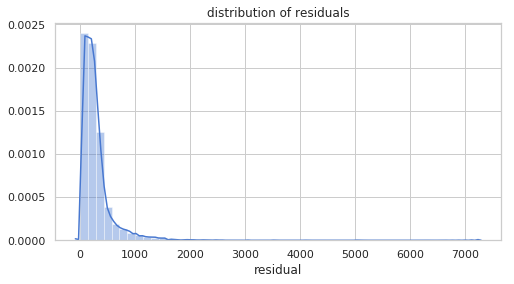# Average residual grouping by ride duration
val_residual = X_val.copy()
val_residual['duration'] = y_val
val_residual['rounded_duration'] = np.around(y_val, -2)
val_residual['residual'] = np.abs(y_val - y_val_pred)
tmp = val_residual.groupby('rounded_duration').mean()
plt.figure(figsize=(8,4))
tmp['residual'].plot()
plt.ylabel('average residual')
plt.title('average residual by ride duration');